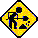# Affine Arithmetic:

## A Correlation-Sensitive Variant of Interval Arithmetic

### Project description

Affine arithmetic (AA) is a self-validated model for numerical computation. Like standard interval arithmetic (IA), it can provide guaranteed bounds for the computed results, taking into account input, truncation, and rounding errors. Unlike interval arithmetic, it keeps track of correlations between computed and input quantities, and is therefore resistant to the catastrophic loss of precision often observed in long interval computations.

In affine arithmetic, a quantity x is represented as a first-degree ("affine") polynomial

x0 + x1 e1 + x2 e2 + ··· + xk ek        (1)
where x0, x1,... xk are known real numbers, and e1, e2,... ek are dummy variables, whose value is only known to be in [-1 .. +1].

Each dummy variable ei represents some source of uncertainty or error in the quantity x --- which may come from input data uncertainty, formula truncation, arithmetic rounding, etc. A dummy variable that appears in two variables x, y implies that their values are partially correlated, i.e. the pair (x, y) ranges over a proper subset of the rectangle (range of x) × (range of y). This information is automatically taken into account by the basic AA operations, so that --- for instance --- the formula (1 + x) + (1 - x) evaluates to an interval of (almost) zero width, even if x has a large uncertainty.

As in standard IA, we can evaluate an arbitrary a mathematical formula in AA by merely replacing each elementary operation or standard function z := f(x,y,...) by a call to the corresponding AA operation. The latter is a procedure that takes affine forms like (1) for the operands x, y, ... and returns the result z in the same format.

If the operation f is itself an affine function of its arguments (which includes addition, subtraction, and multiplication by an exact scalar), then the AA operation can be carried out exactly, except for rounding errors. Otherwise, the quantity f(x,y,...) is not an affine function of the dummy variables ei. In that case, the routine must find a good affine approximation to this function, and introduce a new dummy variable ek to stand for the approximation error.

Affine arithmetic is somewhat similar to Hansen's generalized interval arithmetic , but differs in several important details. For example, in Hansen's model the internal approximation errors are combined with the input uncertainties, whereas in AA they are represented separately---which makes it possible for the approximation error introduced at one step to be canceled out at a later step. Also, in Hansen's model---but not in AA---the joint range of two variables may be a non-convex region.

Affine arithmetic may also be compared to the ellipsoid calculus of Chernousko, Kurzhanski, and Ovseevich [8,9], in that both attempt to record linear correlations between quantities. One basic difference is that in Kurzhanski's model the joint ranges are ellipsoids, whereas in AA they are convex center-symmetric polytopes.

In general, the internal approximation errors of affine arithmetic operations depend quadratically on the width of the input intervals. Therefore, ranges obtained with AA may be substantially more accurate than those obtained with standard IA (whose errors depend linearly on the input range widths).

Obviously, AA operations are more expensive than those of standard IA. However, in many algorithmic contexts (such as zero finding and branch-and-bound optimization) the improved accuracy of AA will generally lead to narrower search ranges and earlier rejection of bad branches. Thus, even though the substituion of AA for IA makes each step more expensive, it may actually reduce the total cost of the algorithm. We are presently collecting concrete problems where this speedup does take place [0, 1, 2, 4, 12].

### Source files:This directory is still under construction. Please excuse the mess!

The following archive files are slightly outdated:

• aa.tar.gz -- Archived with Unix tar/gzip.
• aa.zip -- Archived with PKZip.
• README -- Unpacking and compilation instructions.